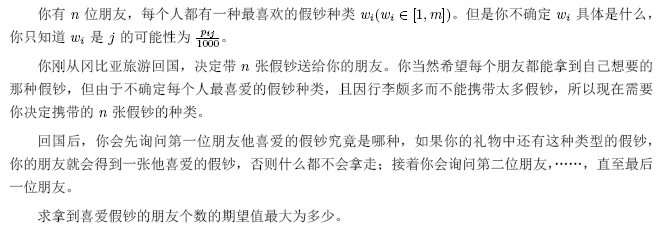# coin

### Decsription数据范围：$n<=3000,m<=300$，保证$\forall i,\sum\limits_{j}p_{ij}=1000$

### Solution

日常期望算不对。。

首先比较明显的一点是，每种类型是独立的，我们可以分开来考虑

先来想一个比较直接的dp

用$a[i][j]$表示第$i$个人最喜欢$j$的概率，记$f[c][i][j]$表示前$i$个人里面恰好有$j$个人最喜爱类型$c$，转移显然：

$f[c][i][j]=f[c][i-1][j]*(1-a[i][j])+f[c][i-1][j-1]*a[i][j]$

再考虑用$g[i][j]$表示第$i$种钞票携带了$j$张，期望拿走的数量，那么有转移：

\begin{aligned} g[i][j]&=\sum\limits_{k=0}^mmin(k,j)*f[i][n][k]\\ &=\sum\limits_{k=0}^j k*f[i][n][k]+\sum\limits_{k=j+1}^mj*f[i][n][k]\\ \end{aligned}

然后我们就可以用一个背包求出答案了，但是这样无论是空间还是时间都很爆炸

​　　考虑$\nabla g[i][j]=g[i][j]-g[i][j-1]$，也就是$\nabla g[i][j]=\sum\limits_{k=j}^m f[i][n][k]$，首先注意到$f[i][n][k]$$i$确定的情况下大小一定是随着$k$的增大而增大的，然后再看回这个$\nabla g[i][j]$，显然这个东西的值应该是非负的，并且当$i$一定，$\nabla g[i][j]$的值随着$j$的增大而单调不升

​　　所以我们可以得到一个结论，当$i$确定的时候，$g[i][j]$是不降的，并且增幅逐渐下降

​

然后又因为每种钞票是独立的，我们可以考虑一个贪心

枚举$n$张钞票的每一张都选什么，对于每一种钞票$c$，我们维护一个隐性的$p_c$（说是“隐性”是因为在实现的时候你并不需要真的去维护这么一个东西），表示钞票$c$当前已经取了$p_c$张，那么从贪心的角度来看，我们当前枚举到的这张钞票，肯定希望新加入这张钞票之后，对应的$g[c]$的增幅最大，所以就可以得到一个大致的流程：从$1$$n$枚举每一张钞票选什么，对于第$i$张钞票，$O(m)$求得最大的$g$的增幅，加入答案，然后对于选择的这个种类$c$，更新其$g$

​　　现在的问题就是怎么维护增幅，为了方便下面只针对确定的$c$类钞票进行表述

一开始的时候$p_c=0$$g[c]$$g[c]$的增幅是很好计算的，$\nabla g[c]=1-\prod\limits_{i=1}^n(1-a[i][c])$，但是$\nabla g[c]$看起来就不能那么直接地进行计算了，所以我们还是看回这个式子：$\nabla g[i][j]=\sum\limits_{k=j}^mf[i][n][k]=1-\sum\limits_{k=0}^{j-1}f[i][n][k]$，那所以我们只要对于第$c$种钞票维护$f[c][i][j]$就好，然后维护一个$sum[c]=\sum\limits_{k=0}^{p_c}f[c][n][k]$，每次更新完$f[c]$之后把$f[c][n][p_c]$加到$sum[c]$里面就好了

​　　接下来就是空间怎么解决：对于$f[c][i][j]$来说，我们是按照$j$进行dp的，显然$j$那维可以滚动掉，$f[c][][j]$$f[c][][j+1]$的更新是$O(n)$的，然后我们就获得了一个$O(nm)$的算法

mark：（弱智操作）谁告诉你听算期望的时候可以钦定顺序的？？？

​

### Code

#include<iostream>
#include<cstdio>
#include<cstring>
using namespace std;
const int N=3010,M=310;
double sum[N],f[M][N];
double a[N][M];
int now[M],pre[M];
int n,m;
double ans;
void dp(int x){
swap(now[x],pre[x]);
int Now=now[x],Pre=pre[x];
f[Now][x]=0;
for (int i=1;i<=n;++i)
f[Now][x][i]=f[Now][x][i-1]*(1-a[i][x])+f[Pre][x][i-1]*a[i][x];
}

int main(){
#ifndef ONLINE_JUDGE
freopen("a.in","r",stdin);
#endif
int x;
scanf("%d%d",&n,&m);
for (int i=1;i<=n;++i)
for (int j=1;j<=m;++j)
scanf("%d",&x),a[i][j]=1.0*x/1000;
for (int i=1;i<=m;++i){
f[i]=1;
for (int j=1;j<=n;++j)
f[i][j]=f[i][j-1]*(1-a[j][i]);
sum[i]=f[i][n];
}
for (int i=1;i<=m;++i) now[i]=0,pre[i]=1;
int which;
double mx;
for (int j=1;j<=n;++j){
mx=0; which=-1;
for (int i=1;i<=m;++i)
if (1-sum[i]>mx)
which=i,mx=1-sum[i];
ans+=mx;
dp(which);
sum[which]+=f[now[which]][which][n];
}
printf("%.10lf\n",ans);
}

posted @ 2018-12-19 21:55  yoyoball  阅读(158)  评论(0编辑  收藏